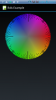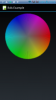# Android QuestionWheel Color Generate

#### Star-Dust

##### Expert
Longtime User
Hello everyone,
I tried to write a code that allows me to draw the attached image on a canvas. But with disappointing results, does anyone know how to give me a hand?or•dost

Longtime User

#### DavideV

##### Active Member
Longtime User
I find a new solutionI think this solution is best

Hi Star-Dust,
B4X:
``````'colored circle generator #1
Private Sub Pattern (Diameter As Int)As Bitmap
Dim Btm As Bitmap
Dim Can As Canvas
Dim Width As Int = Diameter        '200dip
Dim Height As Int = Diameter    '200dip
'Center Point
Dim CenterX As Int = Width/2
Dim CenterY As Int = Height/2
Dim radius As Int = CenterX * CenterY
' RED
Dim redX As Int = Width
Dim redY As Int = Height /2
Dim redRad As Int = Width*Width
' Green
Dim greenX As Int = 0
Dim greenY As Int = Height/2
Dim greenRad As Int = Width*Width
'Blue
Dim blueX As Int = Width/ 2
Dim blueY As Int = Height
Dim blueRad As Int = Width*Width

Btm.InitializeMutable(Width,Height)
Can.Initialize2(Btm)

For i=0 To Width-1
For j=0 To Height-1
Dim a As Int = i - CenterX
Dim b As Int = j - CenterY

Dim distance As Int = a * a + b * b
Dim rdx As Int = i - redX
Dim rdy As Int = j - redY
Dim redDist As Int = (rdx * rdx + rdy * rdy)
Dim redVal As Int = (255 - ((redDist / redRad) * 256))

Dim gdx As Int = i - greenX
Dim gdy As Int = j - greenY
Dim greenDist As Int = (gdx * gdx + gdy * gdy)
Dim greenVal As Int = (255 - ((greenDist / greenRad) * 256))

Dim bdx As Int = i - blueX
Dim bdy As Int = j - blueY
Dim blueDist As Int = (bdx * bdx + bdy * bdy)
Dim blueVal As Int = (255 - ((blueDist / blueRad) * 256))

Dim HSV() As Int=RGBtoHSV(redVal,greenVal,blueVal)
Dim V As Double=HSV(2)/255
Dim c As Int =  Colors.rgb(redVal/v, greenVal/v, blueVal/v)

Can.DrawPoint(i,j,c)
Else
Can.DrawPoint(i,j,Colors.RGB(255,255,255))    'color of points outside the circle
End If
Next
Next
Return Can.Bitmap
End Sub

'RGB to HSV colorspace, probably not accurate
Private Sub RGBtoHSV(red As Int, Green As Int, Blue As Int) As Int()

Dim h,s,v As Int
Dim mMin,mMax As Int
mMin=Min(Min(red,Green),Blue)
mMax=Max(Max(red,Green),Blue)
v=mMax
If v=0 Then
s=0:h=0
Return Array As Int(h,s,v)
End If
s = 255 * (mMax - mMin) / v
If s=0 Then
h=0
Return Array As Int(h,s,v)
End If
If mMax =red Then
h = 0 + 43 * (Green - Blue) / (mMax - mMin)
else if mMax = Green Then
h = 85 + 43 * (Blue - red) / (mMax - mMin)
Else
h = 171 + 43 * (red - Green) / (mMax - mMin)
End If
Return Array As Int(h,s,v)

End Sub``````

and got this:

#### Attachments

•LucaMs and Star-Dust

#### Star-Dust

##### Expert
Longtime User
I realized this ColorDialog
From 15second

•DavideV and LucaMs

#### LucaMs

##### Expert
Longtime User
Sorry..but.... What's aliasing?

Anyway, you would get a better picture but in your case it might be a mistake (question of added pixels).

#### Star-Dust

##### Expert
Longtime UserB4X:
``````Sub ColorWheel As Bitmap
Dim Can As Canvas
Dim Bt As Bitmap
Dim D As Int = 240
Dim Center As Int = D/4
Dim Radius As Int = D/4
Dim X,Y As Int

Bt.InitializeMutable(d/2,d/2)
Can.Initialize2(Bt)
SetAntiAlias(Can)
For h=0 To d
For s=0 To d-10
Can.DrawPoint(x,y ,HSLtoRGB(H,s,100,255))
'            Can.DrawLine(X,Y,X2,Y2,HSLtoRGB(H,s+10,100,255),3)
Next
Next
Return Can.Bitmap
End Sub

Sub HSLtoRGB(Hue As Int, Saturation As Int, Luminance As Int, Alpha As Int ) As Int
Dim temp3(3) As Double , Red As Int, Green As Int, Blue As Int ,temp1 As Double, temp2 As Double ,n As Int
Dim pHue As Double, pSat As Double, pLum As Double , pRed As Double, pGreen As Double, pBlue As Double

pHue = Min(239, Hue) / 239
pSat = Min(239, Saturation) / 239
pLum = Min(239, Luminance) / 239

If pSat = 0 Then
pRed = pLum
pGreen = pLum
pBlue = pLum
Else
If pLum < 0.5 Then
temp2 = pLum * (1 + pSat)
Else
temp2 = pLum + pSat - pLum * pSat
End If
temp1 = 2 * pLum - temp2

temp3(0) = pHue + 1 / 3
temp3(1) = pHue
temp3(2) = pHue - 1 / 3

For n = 0 To 2
If temp3(n) < 0 Then temp3(n) = temp3(n) + 1
If temp3(n) > 1 Then temp3(n) = temp3(n) - 1

If 6 * temp3(n) < 1 Then
temp3(n) = temp1 + (temp2 - temp1) * 6 * temp3(n)
Else
If 2 * temp3(n) < 1 Then
temp3(n) = temp2
Else
If 3 * temp3(n) < 2 Then
temp3(n) = temp1 + (temp2 - temp1) * ((2 / 3) - temp3(n)) * 6
Else
temp3(n) = temp1
End If
End If
End If
Next

pRed = temp3(0)
pGreen = temp3(1)
pBlue = temp3(2)
End If

Red = pRed * 255
Green = pGreen * 255
Blue = pBlue * 255

Return Colors.ARGB(Alpha, Red,Green,Blue)
End Sub

Sub SetAntiAlias (c As Canvas)
Dim r As Reflector
Dim NativeCanvas As Object
r.Target = c
NativeCanvas = r.GetField("canvas")
Dim PaintFlagsDrawFilter As Object
PaintFlagsDrawFilter = r.CreateObject2("android.graphics.PaintFlagsDrawFilter", _
Array As Object(0, 1), Array As String("java.lang.int", "java.lang.int"))
r.Target = NativeCanvas
r.RunMethod4("setDrawFilter", Array As Object(PaintFlagsDrawFilter), Array As String("android.graphics.DrawFilter"))
End Sub``````

#### LucaMs

##### Expert
Longtime User
I know but if you need to pick up a single pixel it might be a pixel added by the antialiasing.

#### LucaMs

##### Expert
Longtime User
Do not forget that there are luckypeople like me: colorblind.
For "us" it is necessary to have the 3 cursors for red, green, blue (and alpha?).

•Peter Simpson and Star-Dust

#### Star-Dust

##### Expert
Longtime User
Do not forget that there are luckypeople like me: colorblind.
For "us" it is necessary to have the 3 cursors for red, green, blue (and alpha?).
You're right, I just saw that it's aesthetically pleasing.

#### Star-Dust

##### Expert
Longtime User
The thread spoke of the color wheel and that was an interesting variant.

Of course you can add the basic color bar

•LucaMs